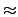Next: 48-bit Linear Congruential Generator Up: Random Number Generators Previous: Random Number Generators

## 64-bit Linear Congruential Generator

This algorithm is the fastest, especially on 64-bit architectures, but does not produce as good a pseudo random number sequence as the prime modulus generators. The next value for the random number state, x[n], is determined from the current value, x[n - 1], by

 x[n] = a x[n - 1] + b   (mod264)   . (3)
The values of the multiplier, a = 2862933555777941757, and the addend, b = 3037000493, are hardwired into the implementation and are known to produce a good random number list. The period of this random number generator is 2641.8 x 1019. This generator is the same as the default one-stream SPRNG  lcg64 generator and has been checked to insure that it satisfies the requirements for maximal period2.

The interface routines for this generator are:

Rng_Type Rng64Seed( Rng_UInt32 i, Rng_UInt32 j );

Rng_Type Rng64Spawn( Rng_Type *x );

int iRng64( Rng_Type *x );

double dRng64( Rng_Type *x );

float fRng64( Rng_Type *x );

This generator is the fastest, particularly on 64 bit computer platforms. It is useful for performance sensitive applications that are insensitive to the pattern content present in the low order bits. As zero is an allowed random number state, this generator is not useful for debugging correct state retirement in a per-particle state implementation.Next: 48-bit Linear Congruential Generator Up: Random Number Generators Previous: Random Number Generators